# Order of Operations: Exponents and Radicals

Author: Sophia Tutorial
##### Description:

Evaluate an expression with exponents or radicals using order of operations.

(more)

Sophia’s self-paced online courses are a great way to save time and money as you earn credits eligible for transfer to many different colleges and universities.*

No credit card required

37 Sophia partners guarantee credit transfer.

299 Institutions have accepted or given pre-approval for credit transfer.

* The American Council on Education's College Credit Recommendation Service (ACE Credit®) has evaluated and recommended college credit for 33 of Sophia’s online courses. Many different colleges and universities consider ACE CREDIT recommendations in determining the applicability to their course and degree programs.

Tutorial
what's covered
1. Order of Operations: Exponents and Radicals

# 1. Order of Operations: Exponents and Radicals

The order of operations outlines an order we should follow to evaluate expressions with multiple operations. We often use PEMDAS to remember the order of operations:

term to know
PEMDAS
An acronym to remember the order of operations: parentheses, exponents, multiplication and division, addition and subtraction.

The "exponents" part of PEMDAS also includes radicals. We don't typically include "radicals" in the acronym, because all radicals can be written as exponents (fractional exponents, specifically). So as long as we remember that exponents and radicals are related, we can remember to evaluate both exponents and radicals after parentheses, but before multiplication, division, addition, and subtraction.

Let's focus first on evaluating expressions containing exponents: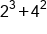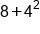Evaluate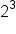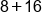Evaluate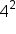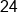Our Solution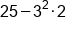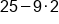Evaluate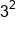first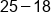Then multiply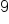by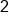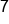Our Solution

When we encounter radicals (roots, such as square roots or cube roots), we treat them as being on the same level as exponents in the order of operations, and evaluate them before multiplication, division, addition and subtraction. This is illustrated in the examples below: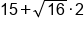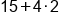Evaluate the square root first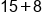Then multiply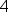by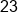Our Solution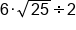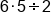Evaluate the square root first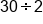Then multiply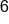by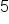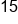Our Solution

What do we do when an expression contains both exponents and radicals? Because exponents and radicals are at the same level according to the order of operations, we can think of their relationship being like multiplication and division, or addition and subtraction: we evaluate exponents and radicals as we see them reading left to right.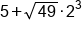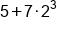Evaluate the square root first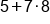Then evaluate the exponent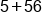Multiplyby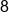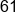Our Solution

summary
When following order of operations, exponents and radicals (roots, such as square roots and cube roots) are considered at the same time. This means that we must evaluate all exponents and all radicals before moving on to the remaining operations in an expression, following the order of operations.

Rating